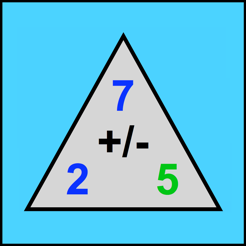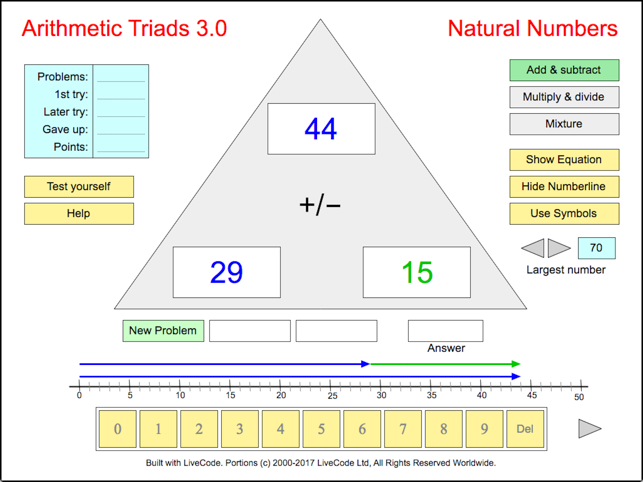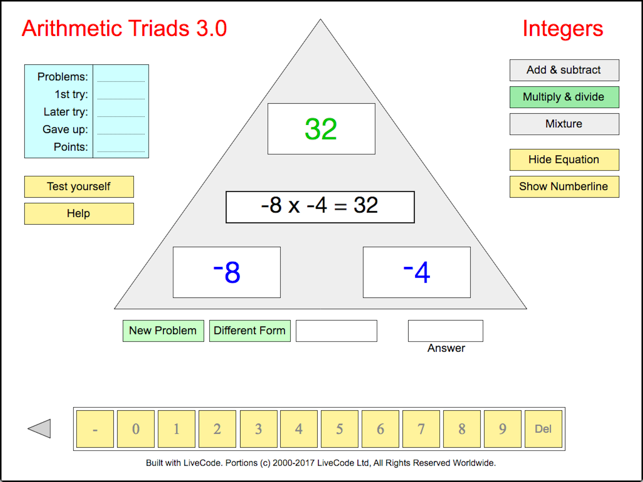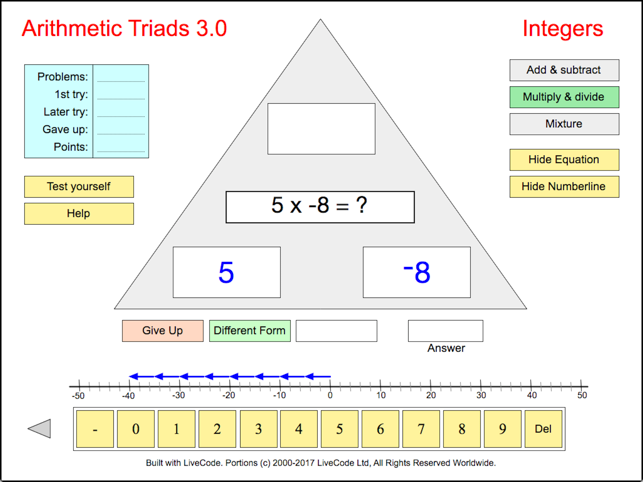•••## Description

This app gives students a chance to improve their basic arithmetic skills as they solve problems involving the addition, subtraction, multiplication, and division of integers. It emphasizes the relationships between the different operations. 7+5=12 means the same thing as 12-7=5, for example, and 5x3 equals both 5+5+5 and 3+3+3+3+3. Each problem is presented simply as two given numbers and a blank, an equation that includes a question mark, and/or graphically as arrows along a number line. Once a problem has been solved, the student has the option of seeing the same basic math fact in other forms. 100 is the largest number that appears in any of the problems, but that maximum number can be adjusted downward. Students can solve the problems just for practice, or test themselves and earn points based on their level of success.

Version 3.11

Bug fixes

## Information

Seller
Thomas Bretl
Size
33.8 MB
Category
Education
Compatibility

Requires iOS 8.0 or later. Compatible with iPad.

Languages

English

Age Rating
4+
•